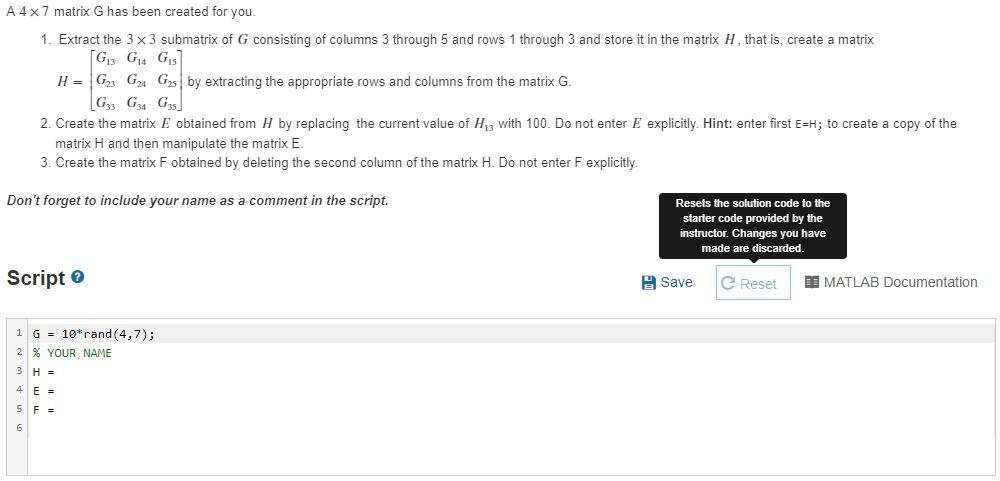# Question A 4x7 matrix G has been created for you. 1. Extract the 3 x 3 submatrix of G consisting of columns 3 through 5 and rows 1 through 3 and store it in the matrix H that is, create a matrix [G GA G H = G23 G24 Gs by extracting the appropriate rows and columns from the matrix G. G3 G3 GS 2. Create the matrix E obtained from H by replacing the current value of His with 100. Do not enter E explicitly. Hint: enter first E=H; to create a copy of the matrix H and then manipulate the matrix E. 3. Create the matrix F obtained by deleting the second column of the matrix H. Do not enter F explicitly. Don't forget to include your name as a comment in the script. Resets the solution code to the starter code provided by the instructor. Changes you have made are discarded. Script Save e Reset BI MATLAB Documentation 1 G = 10*rand (4,7); 2 % YOUR NAME 3 HD 4 E- 5 F = 6OBQQXS The Asker · Computer ScienceTranscribed Image Text: A 4x7 matrix G has been created for you. 1. Extract the 3 x 3 submatrix of G consisting of columns 3 through 5 and rows 1 through 3 and store it in the matrix H that is, create a matrix [G GA G H = G23 G24 Gs by extracting the appropriate rows and columns from the matrix G. G3 G3 GS 2. Create the matrix E obtained from H by replacing the current value of His with 100. Do not enter E explicitly. Hint: enter first E=H; to create a copy of the matrix H and then manipulate the matrix E. 3. Create the matrix F obtained by deleting the second column of the matrix H. Do not enter F explicitly. Don't forget to include your name as a comment in the script. Resets the solution code to the starter code provided by the instructor. Changes you have made are discarded. Script Save e Reset BI MATLAB Documentation 1 G = 10*rand (4,7); 2 % YOUR NAME 3 HD 4 E- 5 F = 6
More
Transcribed Image Text: A 4x7 matrix G has been created for you. 1. Extract the 3 x 3 submatrix of G consisting of columns 3 through 5 and rows 1 through 3 and store it in the matrix H that is, create a matrix [G GA G H = G23 G24 Gs by extracting the appropriate rows and columns from the matrix G. G3 G3 GS 2. Create the matrix E obtained from H by replacing the current value of His with 100. Do not enter E explicitly. Hint: enter first E=H; to create a copy of the matrix H and then manipulate the matrix E. 3. Create the matrix F obtained by deleting the second column of the matrix H. Do not enter F explicitly. Don't forget to include your name as a comment in the script. Resets the solution code to the starter code provided by the instructor. Changes you have made are discarded. Script Save e Reset BI MATLAB Documentation 1 G = 10*rand (4,7); 2 % YOUR NAME 3 HD 4 E- 5 F = 6